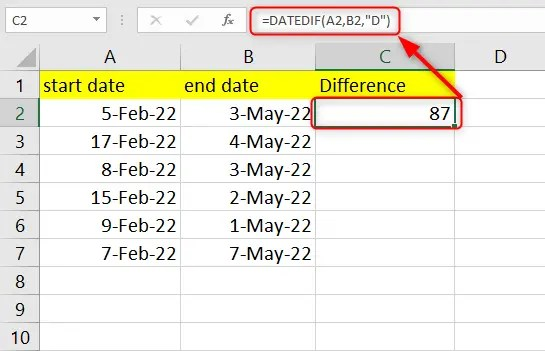# How to minus dates in Excel-3 easy methods

Microsoft Excel is one of the most reliable spreadsheet programs when it comes to dealing with data. You can perform a lot of mathematical computations using the different functions in Excel. One of the functions Excel offers is the DATE function, where you can calculate the additions and differences between dates. You can minus or add days to dates, find out the differences between dates, and even find a future or past date.

The guide below gives you the steps you can take to minus in Excel dates. Let’s get started.

## Steps to take to calculate the differences between two dates in Excel

You can use the DATEDIF function when you want to calculate the difference between two dates in Excel. Your formula will be like this:

=DATEDIF( Start_date, End_date “unit”)

With this function, the Start date has to be lesser than the End date. In cases where the start date is greater, Excel will return the result as #NUM!

The unit stands for the unit of measure, which can be a day (D), month (M), or year(Y). It is not in the function syntax, so you will have to type it yourself.

1. In an open Excel worksheet, click on a cell where you want to apply your results.

3. Next, click on the end date and start date cell references.

4. Click the Enter key to get your results.5. To apply the formula to other cells, use the AutoFill handle.

## Steps to take to minus days from a date in Excel

You can use minus days from a date in Excel by simply subtracting two cell values.

1. Enter your initial date values in the first column in an open Excel workbook.

2. Enter the days you wish to subtract from your date in the second column. make sure you put a minus sign before your values.

3. Next, click a cell in column C where you want to display your result. Enter =A2 + B2 in this cell and press the Enter key.4. Use the AutoFill handle to copy down the formula to the other cells.

## Steps to take to subtract months from a specific date in Excel

To minus a month or number of months from a specific date in Excel, you will need to use the EDATE function. The Functions syntax is:

`=EDATE(start_date, months)`

1. In an open Excel worksheet, click on a cell where you want to display your results.

3. Next, click on the start date cell reference.

4. Next, click on the cell referencing the months to delete. Remember to enter a negative number (place a minus symbol before the months).

5. Click the Enter key to get your results.6. To apply the formula to other cells, use the AutoFill handle.

Notes:

Excel may display serial numbers as results depending on the cell format of your formula cells. You can easily change the format to be in Date format from the Format Cells dialog window.

To access the dialog window, click Ctrl + 1, which launches Format Cells  window. In the Category section, click on the Date option and select a format that you want.